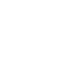# Order Form Calculator

You can add a customizable calculation form based on the selected parameters. To do this, add a "Calculation field" to vertical forms with multiple inputs. You can set up calculation parameters in the Quantity, Dropdown, Checkbox, and One-line input fields.
How it works
— Add a vertical form with multiple inputs from the "Form" category, e.g. BF204N, BF310N, BF311N, BF402N, BF403N, or BF502N.
— Create input fields, e.g. width, length.
— Assign a unique variable name to each field, e.g. "width" and "length."
— Type in a calculation formula using mathematical operators such as +, -, *, /, () (e.g. "width*length")
— When the customer fills out the form, calculations will be performed automatically, the result will appear in the field.
Example
A simple calculator to estimate the cost of a tabletop based on length and width.

Set the dimensions of the tabletop to find out the final price
Enter tabletop length in meters, from 1 to 5
Enter tabletop width in meters, from 1 to 5
Total Price
0\$

How to create a form like the one in the example
Add a vertical form with multiple inputs to the page.
Block Library → Form → BF204N.Open the Content panel of the block by clicking on the orange button in the top left corner of the block. Add a title and description in the Block Header tab.

Create a field for a tabletop's length:
— Add a One line input field.
— Set a variable name. We've chosen length for this example.

Create a width field using the same method.
Create a field where the total cost of the tabletop will be calculated automatically:
— Add a title, subtitle, and variable name;
— Provide a cost calculation formula.

The formula used in the example is length*width*160, where 160 is the price per square meter.
Use only simple mathematical operators: +, -, *, /, and ().
Example of a complex order form calculator
An order form calculator with Checkbox and Dropdown fields.
There are 5 parameters that will determine the final price:
Material — type of wood
Length — tabletop length
Width — tabletop width
Cover — tabletop coating
Legs — type of table legs

The cost calculation formula: length*width*(material+cover)+legs.

To calculate the final price, set the following values: type of wood, size, coating, and type of legs.
Choose wood type
Select length in meters, from 1 to 5
1
5
Select width in meters, from 1 to 5
0.5
3
Price
0\$